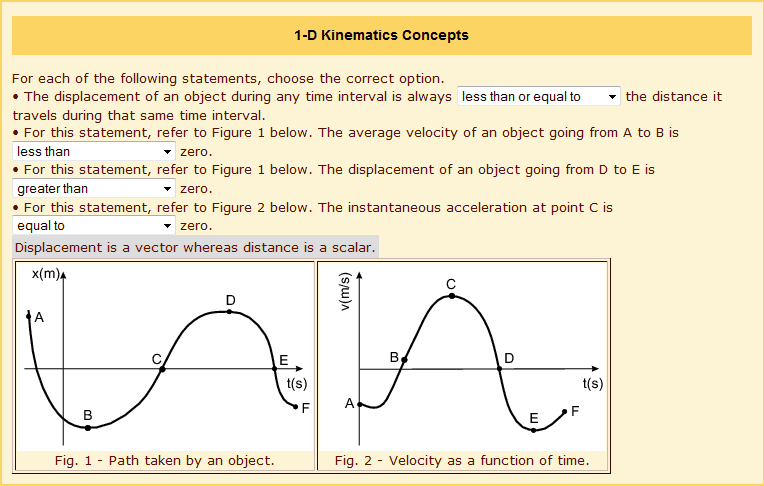# 1-D Kinematics

Here is my attempt at the problem:I have no idea which one is wrong (I know they all aren't... at least I hope not).

Simon Bridge
Homework Helper
So - how would you go about figuring which one is wrong?
Explain your reasoning for each one, and see if there is anything inconsistent.
Remember - we cannot do your homework for you.

ah god, I remember the displacment vs distance thing -.-
don't worry though, distance becomes arc length and displacment becomes $r-r_0$ later on, far more intuative, at least for meI think your problem isn't a lack of understanding but one of looking at the wrong graphs, I remember I hated these type of things in my early studies as well

As Simon says, go through your reasoning for each answer, write it down and go over it
if you do that it should become apparent where your problem is

Simon Bridge
Homework Helper
Also tell us about it - that way we can be of most use.
I can see the blind-spot you have, but it is something you'll see by going carefully step-by-step :)

@genericusrnme
It is conceptually easier in more than one dimension isn't it?
But it's mathematically trickier so...

I think what I have is wrong is the instantaneous acceleration. At C, if the velocity starts to go down, then it must be decelerating... which means instantaneous acceleration would be less than 0.

Simon Bridge
Homework Helper
What property of the v-t graph gives the instantaneous acceleration?

Remember - we cannot do your homework for you, and that includes indirectly via a fishing expedition.
So what we have to do is try to guide your reasoning ... which we cannot do if you don't tell us.

- Displacement will always be less than or equal to distance traveled because displacement is just the shortest distance between an initial point and the resting point. So, if you take an exact straight line, distance traveled will be equal to displacement. But, you can also take any other path and it will obviously be greater than the displacement.

- The average velocity of an object going from A to B should be greater than 0 because it spends more time increasing on that interval than decreasing. What tripped me up there was the fact that it was on the negative side of the graph for a large portion of that interval. I'm still unsure about that one.

- Unless point D and E shared the same coordinates, the displacement will always be greater than 0.

- At C, if the velocity starts to go down, then it must be decelerating... which means instantaneous acceleration would be less than 0.

I'm not quite sure what property of the v-t graph would give the instantaneous acceleration. :(

Simon Bridge
Homework Helper
Lets see if we cannot clear up your thinking a bit:

The magnitude of the displacement is the distance between the endpoints.
The displacement itself is this magnitude multiplied by the unit vector pointing from start to finish.
It is a vector. Since displacement is a vector, it can be negative.
In fact, you will notice that the displacement-time graph has negative displacements on it.

average velocity = change in displacement over change in time.
velocity is also a vector.

average acceleration = change in velocity over change in time.
acceleration is also a vector.

"change in" is "final minus initial".
so if the final is less than the initial, the change is negative.

you can think of displacement as a change in position.

The instantaneous acceleration is the slope of the tangent to the v-t graph at the point.
The displacement is the area under the v-t graph.
These are probably the most important things to remember, particularly for kinematics.

slowing down forwards and speeding up backwards are both negative accelerations.

OK so try again, only using these terms.

Last edited:
Thank you for bearing with me.

- The average velocity from A to B will be negative because V = change in displacement / change in time and the change in displacement will be negative.

- Then, for the displacement from D to E, it should be less than zero because the final is less than the initial and we can think of displacement as a "change in" position.

- Lastly, the slope of the tangent at that point on the line would be 0 so be 0, so the instantaneous acceleration is definitely 0.

Simon Bridge
Homework Helper
Well done - having gone through that, you should be able to see which one of your original answers was incorrect :)

Notice that from C to E on the v-t graph, the object starts out moving to the right (+x direction) quite fast, is slowing at a near-constant rate as it approaches D where it changes direction and picks up speed, to the left this time, at the same rate as it was losing speed before.

That's the last bit where people tend to trip up.

The main lesson I want to draw out for you is this: don't be scared of being wrong.
Nobody likes exposing their thoughts/reasoning to strangers - what if they are silly and they make us feel bad?
If I had just replied directly to your question, the deeper misunderstandings would not have come to the surface and you'd end up confused even more.
By risking being wrong you got to (a) solve your problem yourself, and (b) see how much of what you were doing was actually correct after all.
The reward is usually worth the risk - especially in science where everyone is wrong almost all the time anyway :)

Last edited:
No, trust me. I'd much rather understand the concepts. All of this stuff is built on, so if I don't get the concepts now, I will feel the pain later in the semester.

Simon Bridge